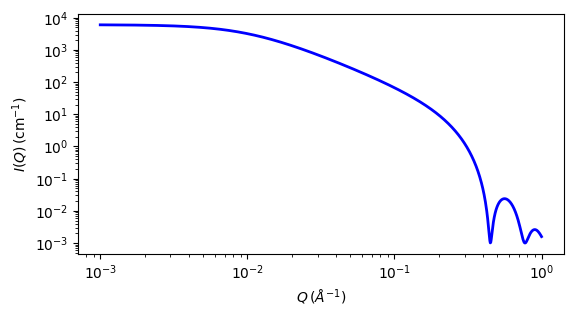# mass_fractal

Mass Fractal model

Parameter

Description

Units

Default value

scale

Scale factor or Volume fraction

None

1

background

Source background

cm-1

0.001

10

fractal_dim_mass

Mass fractal dimension

None

1.9

cutoff_length

Cut-off length

100

The returned value is scaled to units of cm-1 sr-1, absolute scale.

Calculates the scattering from fractal-like aggregates based on the Mildner reference.

Definition

The scattering intensity $$I(q)$$ is calculated as

$I(q) = scale \times P(q)S(q) + background$
$P(q) = F(qR)^2$
$F(x) = \frac{3\left[sin(x)-xcos(x)\right]}{x^3}$
$S(q) = \frac{\Gamma(D_m-1)\zeta^{D_m-1}}{\left[1+(q\zeta)^2 \right]^{(D_m-1)/2}} \frac{sin\left[(D_m - 1) tan^{-1}(q\zeta) \right]}{q}$
$scale = scale\_factor \times NV^2(\rho_\text{particle} - \rho_\text{solvent})^2$
$V = \frac{4}{3}\pi R^3$

where $$R$$ is the radius of the building block, $$D_m$$ is the mass fractal dimension, $$\zeta$$ is the cut-off length, $$\rho_\text{solvent}$$ is the scattering length density of the solvent, and $$\rho_\text{particle}$$ is the scattering length density of particles.

Note

The mass fractal dimension ( $$D_m$$ ) is only valid if $$1 < mass\_dim < 6$$. It is also only valid over a limited $$q$$ range (see the reference for details).Fig. 110 1D plot corresponding to the default parameters of the model.

Source

mass_fractal.py $$\ \star\$$ mass_fractal.c $$\ \star\$$ sas_gamma.c $$\ \star\$$ sas_3j1x_x.c

References

1. D Mildner and P Hall, J. Phys. D: Appl. Phys., 19 (1986) 1535-1545 Equation(9)

Authorship and Verification

• Author: